Orginal Article

# A DUAL-LEVEL SINGULAR BOUNDARY METHOD FOR LARGE-SCALE HIGH FREQUENCY SOUND FIELD ANALYSIS

State Key Laboratory of Hydrology-Water Resources and Hydraulic Engineering & Center for Numerical Simulation Software in Engineering and Sciences, College of Mechanics and Materials, Hohai University, Nanjing 211100, China

Abstract

Numerical simulation of the large-scale high frequency sound field is a computational challenging task. To solve the difficulty that the traditional boundary collocation methods are not easy to be applied to large-scale problems thanks to the resulting large-scale fully-populated matrix, a dual-level singular boundary method is proposed in this study. By introducing a dual level structure, the fully-populated matrix is transformed to a large-scale locally supported sparse matrix on fine mesh. The bottleneck of excessive storage requirements and a large number of operations encountered by the traditional singular boundary method is hereby avoided. Secondly, the method uses only coarse mesh nodes to evaluate far-field contributions, and it is a kernel-independent algorithm. In comparison with the fast multipole method, the dual-level singular boundary method performs higher adaptability and flexibility. In addition, the dual level structure plays a role of preconditioner, which makes the method is very efficient for solving matrix with large scale, high rank and high condition number. In scattering sphere example, the dual-level singular boundary method simulates well the acoustic scattering problem with up to dimensionless wavenumber of 160 when the number of degrees of freedom is taken as 100 000. In the benchmark human head sound scattering, the dual-level singular boundary method using 80 000 degrees of freedom performs 78.13% faster than the COMSOL, and it is noted that the computational frequency is up to 25 kHz, which is far beyond the limit of hearing of human ear.

Keywords： singular boundary method ; high frequency sound field analysis ; modified dual-level algorithm ; meshless method ; origin intensity factor

0

Li Junpu, Chen Wen. A DUAL-LEVEL SINGULAR BOUNDARY METHOD FOR LARGE-SCALE HIGH FREQUENCY SOUND FIELD ANALYSIS[J]. Acta Mechanica Sinica, 2018, 50(4): 961-969 https://doi.org/10.6052/0459-1879-18-100

## 1 双层奇异边界法技术路线

### 1.1 奇异边界法基本公式回顾

$∇2ϕ(x)+k2ϕ(x)=0,∀x∈Ω$

$ϕ(x)=ϕ̅(x),∀x∈S1(Dirichlet boundary condition)$

$q(x)=q̅(x),∀x∈S2(Neumann boundary condition)$

$\begin{equation*}\left.\begin{split}&G(x,y)=\dfrac{{\rm e}^{{\rm i}kr}}{4{π}r}\\&Q(x,y)=\dfrac{\partial G(x,y)}{\partial n(x)}=\\&\quad\quad\quad\dfrac{{\rm e}^{{\rm i}kr}}{4{π}r^3}({\rm i}kr-1)\big\langle(x,y)\cdot n(x)\big\rangle\end{split}\right\}\tag*{(4)}\end{equation*}$

$ϕ(xi)=∑j=1N‍λjG(xi,yj)$

$q(xi)=∑j=1N‍λjQ(xi,yj)$

$ϕ̅(xi)=∑j=1≠iN‍λjG(xi,yj)+λiG(xi,yi)$

$q̅(xi)=∑j=1≠iN‍λjQ(xi,yj)+λiQ(xi,yi)$

$Aλ=b$

### 1.2 双层奇异边界法基本思想

(1)细网格上的满阵被转化为稀疏矩阵, 克服了边界型离散方法的过度存储需求和过高计算负荷;

(2)最终需要求解的矩阵是一个细网格上的稀疏矩阵, 双层结构起到了预调节的作用;

(3)双层奇异边界法仅通过粗网格计算远场相互作用, 提高了奇异边界法的计算效率.

### 1.3 双层奇异边界法逻辑步骤

Table 1   Nomenclature in the dual-level singular boundary method

$CΩ2$sparse matrix on the fine mesh
$Ω1$coarse mesh subscript
$Ω2$fine mesh subscript
$I+$positive projection operator
$I-$negative projection operator
$Tol$preset convergence criterion
*$r0$characteristic radius of range of influence of near
field region

$AΩ1λΩ10=bΩ1$

$λΩ20=I+λΩ10$

$VΩ20=bΩ2-AΩ2λΩ20$

$CΩ2αΩ20=VΩ20$

$γΩ20=αΩ20+λΩ20$

$\begin{equation*}Rerr^0_{\varOmega_2}=\sqrt{\sum^{N_{\varOmega_2}}_{i=1}\Bigg|b^i_{\varOmega_2}-\sum^{N_{\varOmega_2}}_{j=1}A^{ij}_{{\varOmega_2}}\bigg(\gamma^0_{\varOmega_2}\bigg)_j\Bigg|^2\Bigg/\sum^{N_{\varOmega_2}}_{i=1}\Big|b^i_{\varOmega_2}\Big|^2}\tag*{(15)}\end{equation*}$

$k$次循环后, 假设 $γΩ2k$已求得.

$χΩ2k=bΩ2-AΩ2γΩ2k$

$χΩ1k=I-χΩ2k$

$AΩ1αΩ1k=χΩ1k$

$λΩ2k+1=γΩ2k+I+αΩ1k$

$VΩ2k+1=bΩ2-AΩ2λΩ2k+1$

$CΩ2αΩ2k+1=VΩ2k+1$

$γΩ2k+1=αΩ2k+1+λΩ2k+1$

$RerrΩ2k+1=∑i=1NΩ2‍|bΩ2i-∑j=1NΩ2‍AΩ2ij(γΩ2k+1)j|2/∑i=1NΩ2‍|bΩ2i|2$

## 2 数值实验

$Error=∑i=1NT‍|u(i)-u̅(i)|2/∑i=1NT‍|u̅(i)|2$

$Rerr=∑i=1N‍|bi-∑j=1N‍Aijλj|2/∑i=1N‍|bi|2$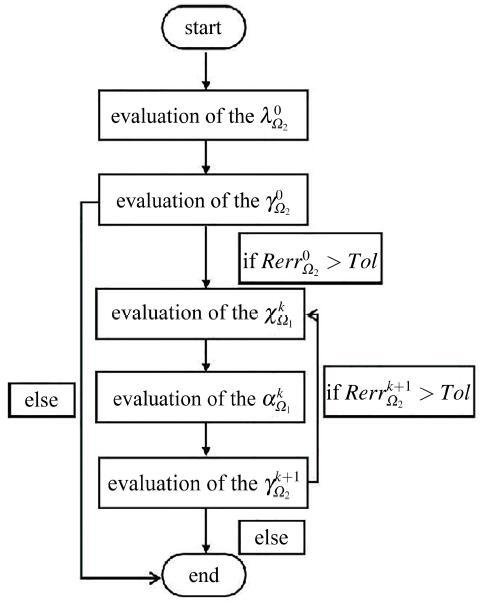Fig.1   The block diagram of the dual-level singular boundary method

$ϕS=∑l=0N‍χlhl(kr)Pl(cosθ)$

$ϕI+ϕS=0,at(x,y,z)∈S$

$χl=-u0(2l+1)iljl(ka)hl(ka),a=1$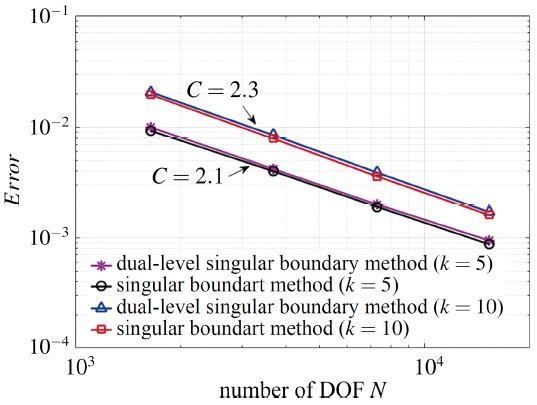Fig.2   Convergence of the dual-level singular boundary method and the singular boundary method

Table 2   CPU time of the dual-level singular boundary method (unit: s)

Nodes16463686729115 284
CPU($k=5$)2.95.918.3109.5
CPU($k=10$)5.610.529.3146.3

Table 3   CPU time of the singular boundary method (unit: s)

Nodes16463686729115 284
CPU($k=5$)0.41.76.128.1
CPU($k=10$)0.42.415.134.8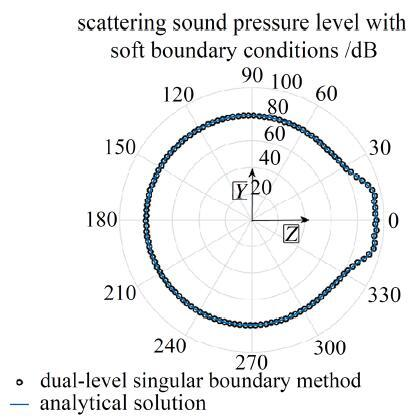Fig.3   Polar diagram of the acoustic scattering characteristics of scattering sphere

$∂ϕS∂n+∂ϕl∂n=0,atr=a$

$χl=-ϕ0(2l+1)illjl-1(ka)-(l+1)jl+1(ka)lhl-1(ka)-(l+1)hl+1(ka)$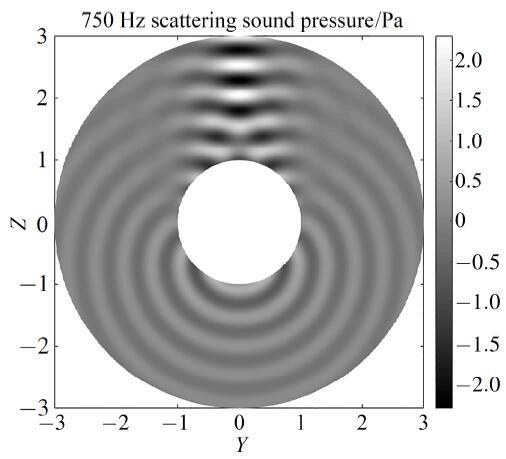Fig.4   Scattered sound pressure evaluated by the dual-level singular boundary method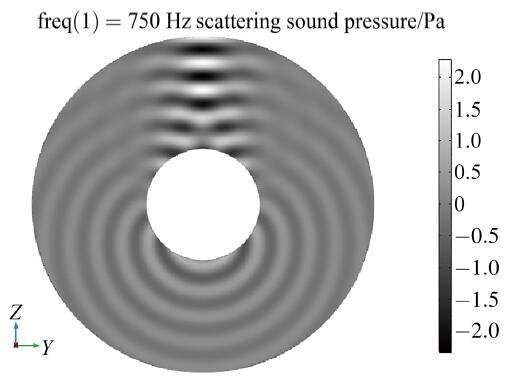Fig.5   Scattered sound pressure evaluated by COMSOL

$SPL=20lg[p(e)/pref]$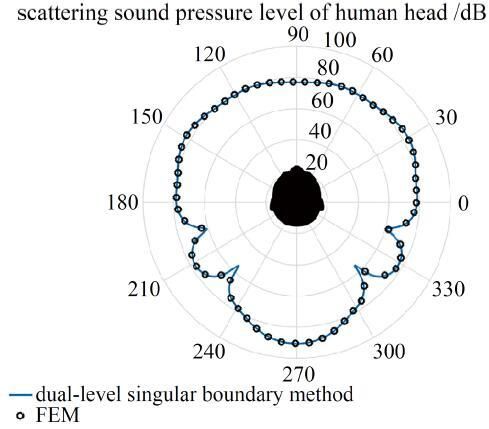Fig.6   Polar diagram of the acoustic scattering characteristics of human head model

## 附录 1

$∫S‍G0(x,y)q(y)-∂G0(x,y)∂ne(y)ϕ(y)dS(y)=0,∀x∈Ωe$

$∂G0(xi,yi)∂ne(yj)=-1Ai∑j=1≠iN‍∂G0(xi,yi)∂ne(yj)Aj,∀xi∈S$

$limyj→xi∂G0(xi,yj)∂ne(xi)+∂G0(xi,yj)∂ne(yj)=0$

$∂G0(xi,yi)∂ne(xj)=1Ai∑j=1≠iN‍∂G0(xi,yj)∂ne(yj)Aj,∀xi∈S$

$f(r)=12r2$

$ϕ(yj)=∂f(yj-xi)∂ne(xi)=〈(yj-xi)⋅ne(xi)〉$

$q(yj)=∂ϕ(yj)∂ne(yj)=〈ne(xi)⋅ne(yj)〉$

$G0(xi,yi)=-1Ai⋅∑j=1≠iN‍[G0(xi,yj)〈ne(xi)⋅ne(yj)〉-$

$∂G0(xi,yj)∂ne(yj)〈(yj-xi)⋅ne(xi)〉]Aj,∀xi∈S$

$G(xi,yi)=G0(xi,yi)+k4<$

$∂G(xi,yi)∂ne(xi)=∂G0(xi,yi)∂ne(xi),r→0$

https://doi.org/10.13140/RG.2.2.13247.00162.

The authors have declared that no competing interests exist.

## 参考文献 原文顺序 文献年度倒序 文中引用次数倒序 被引期刊影响因子

  刘璟泽, 姜东, 韩晓林等. 曲线加筋Kirchhoff-Mindlin板自由振动分析. 力学学报, 2017, 49(4): 929-939 (Liu Jingze, Jiang Dong, Han Xiaolin, et al.Free vibration analysis of curvilinearly stiffened Kirchhoff-Mindlin plates.Chinese Journal of Theoretical and Applied Mechanics, 2017, 49(4): 929-939 (in Chinese))  Wang XM, Liu S, Liu ZP.Underwater sonar image detection: A combination of non-local spatial information and quantum-inspired shuffled frog leaping algorithm.Plos One, 2017, 12(5): 1-30  Li JP, Chen W.Singular boundary method based on time-dependent fundamental solutions for active noise control.Numerical Methods for Partial Differential Equations, 2018, 34(4): 1401-1421  刘硕, 方国东, 王兵等. 近场动力学与有限元方法耦合求解热传导问题. 力学学报, 2018, 50(2): 339-348 (Liu Shuo, Fang Guodong, Wang Bing, et al.Study of thermal conduction problem Using coupled peridynamics and finite element method.Chinese Journal of Theoretical and Applied Mechanics, 2018, 50(2): 339-348 (in Chinese))  Zhang JM, Lin WC, Dong YQ, et al.A double-layer interpolation method for implementation of BEM analysis of problems in potential theory.Applied Mathematical Modelling, 2017, 51: 250-269  Li XL, Li SL.Improved complex variable moving least squares approximation for three-dimensional problems using boundary integral equations.Engineering Analysis with Boundary Elements, 2017, 84: 25-34  高效伟, 刘健, 彭海峰. 集成单元边界元法及其在主动冷却热防护系统分析中的应用. 力学学报, 2016, 48(4): 994-1003 (Gao Xiaowei, Liu Jian, Peng Haifeng.Integrated unit bem and its application in analysis of actively cooling tps.Chinese Journal of Theoretical and Applied Mechanics, 2016, 48(4): 994-1003 (in Chinese))  Gao XW, Li ZY, Yang Kai, et al.Element differential method and its application in thermal-mechanical problems.International Journal for Numerical Methods in Engineering, 2018, 113(1): 82-108  Li XL, Li SL.Analysis of the complex moving least squares approximation and the associated element-free Galerkin method.Applied Mathematical Modelling, 2017, 47: 45-62  Li JP, Chen W.A modified singular boundary method for three-dimensional high frequency acoustic wave problems.Applied Mathematical Modelling, 2018, 54: 189-201  Li JP, Chen W, Gu Y.Error bounds of singular boundary method for potential problems.Numerical Methods for Partial Differential Equations, 2017, 33(6): 1987-2004  杨建军, 郑健龙. 无网格局部强弱法求解不规则域问题. 力学学报, 2017, 49(3): 659-666 (Yang Jianjun, Zheng Jianlong.Meshless local strong-weak (MLSW) method for irregular domain problems.Chinese Journal of Theoretical and Applied Mechanics, 2017, 49(3): 659-666 (in Chinese))  Shen L, Liu YJ.An adaptive fast multipole boundary element method for three-dimensional acoustic wave problems based on the Burton-Miller formulation.Computational Mechanics, 2007, 40(3): 461-472  Li WW, Chen W, Fu ZJ.Precorrected-FFT accelerated singular boundary method for large-scale three-dimensional potential problems.Communications in Computational Physics, 2017, 22(2): 460-472  Cui TJ, Weng CC, Chen G, et al.Efficient MLFMA, RPFMA, and FAFFA algorithms for EM scattering by very large structures.IEEE Transactions on Antennas & Propagation, 2004, 52(3): 759-770  Li JP, Chen W, Fu ZJ. A modified dual-level algorithm for large-scale three-dimensional Laplace and Helmholtz equation. Computational Mechanics, 2018, https://doi.org/10.1007/s00466-018-1536-2  陈文, 李珺璞, 傅卓佳. 基于时间依赖基本解的奇异边界法模拟二维狄利克雷边界标量波方程. 计算力学学报, 2017, 34(2): 231-237 (Chen Wen, Li Junpu, Fu ZhuoJia. Singular boundary method based on time-dependent fundamental solution for 2D scalar wave equation.Chinese Journal of Computational Mechanics, 2017, 34(2): 231-237 (in Chinese))  Fu ZJ, Chen W, Gu Y.Burton-Miller-type singular boundary method for acoustic radiation and scattering.Journal of Sound & Vibration, 2014, 333(16): 3776-3793  Mccowen A.Efficient 3-D moment-method analysis for reflector antennas using a far-field approximation technique.IEE Proceedings-Microwaves, Antennas and Propagation, 1999, 146(1): 7-12  Chen JT, Lee YT, Lin YJ.Analysis of mutiple-shepers radiation and scattering problems by using a null-field integral equation approach.Applied Acoustics, 2010, 71(8): 690-700  Liu L.Single layer regularized meshless method for three dimensional exterior acoustic problem.Engineering Analysis with Boundary Elements, 2017, 77: 138-144  谷岩, 陈文. 改进的奇异边界法模拟三维位势问题. 力学学报, 2012, 44(2): 351-360 (Gu Yan, Chen Wen.Improved singular boundary method for three dimensional potential problems.Chinese Journal of Theoretical and Applied Mechanics, 2012, 44(2): 351-360 (in Chinese))  Liu L, Zhang H.Single layer regularized meshless method for three dimensional Laplace problem.Engineering Analysis with Boundary Elements, 2016, 71: 164-168  王俊鹏, 校金友, 文立华. 大规模边界元模态分析的高效数值方法. 力学学报, 2017, 49(5): 1070-1080 (Wang Junpeng, Xiao Jinyou, Wen Lihua.An efficient numerical method for large-scale modal analysis using boundary element method.Chinese Journal of Theoretical and Applied Mechanics, 2017, 49(5): 1070-1080 (in Chinese))  Li JP, Fu ZJ, Chen W.Numerical investigation on the obliquely incident water wave passing through the submerged breakwater by singular boundary method.Computers & Mathematics with Applications, 2016, 71(1): 381-390/

 〈〉